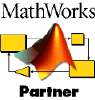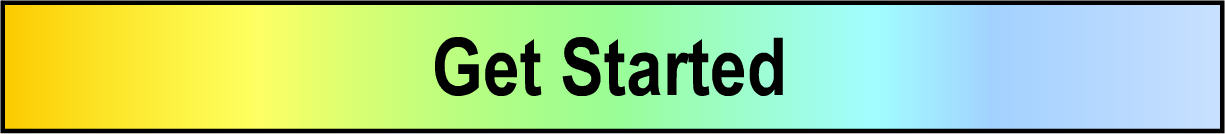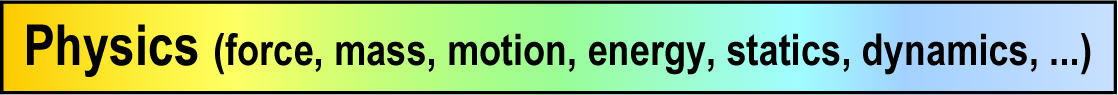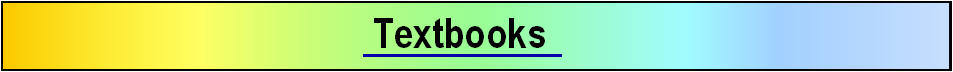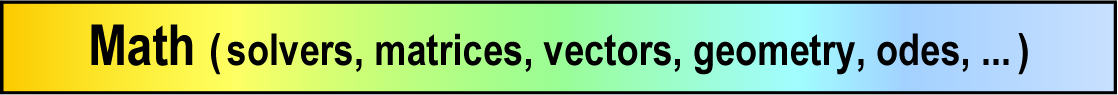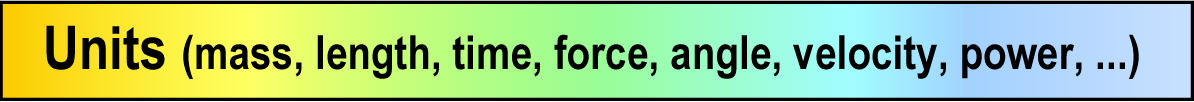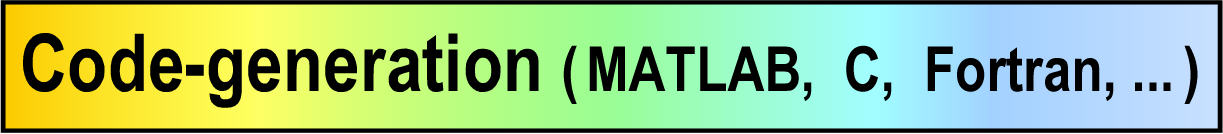Force, mass, motion, and energy

Vectors, tensors, and geometry
• Commands for calculating angles, distances, and unit vectors.
• Creates vectors and dyadics (tensors) through special constructors.
• Multi-basis capable dot-products and cross-products.
• Calculates ordinary and partial derivatives of vectors (accounts for change in both magnitude and direction)
• Uses the symbol   >   to clearly distinguish vectors from scalars.
• Built-in zero vector, zero dyadic, and unit dyadic, i.e.,   0>   0>>   1>>
Rotational kinematics
• Easy-to-use Rotate command sets rotation matrix, angular velocity, angular acceleration.
• Individual commands to SetRotationMatrix, SetAngularVelocity, or SetAngularAcceleration,
• Automatically calculates rotation matrices, angular velocities, and angular accelerations.
• Commands for calculating angles between vectors or unit vectors.
• Forward kinematics, inverse kinematics, and mixed problems.
Translational kinematics
• Easy-to-use Translate command sets position, velocity, acceleration.
• Individual commands to SetPosition, SetVelocity, or SetAcceleration,
• Automatically calculates position vectors, velocities, and accelerations.
• Commands for calculating distance (length) and speed.
• Forward kinematics, inverse kinematics, and mixed problems.
Statics and dynamics   (Newton/Euler, D'Alembert, and Kane)
• GetStatics sums forces and moments for static ("free-body") analysis.
• GetDynamics automates Newton/Euler (D'Alembert) dynamics.
• GetStaticsKane and GetDynamicsKane eliminate constraint forces for efficient analyses.
• Forms linear momentum, angular momentum, and kinetic energy.
• Employs highly efficient algorithms to formulate and solve equations of motion.
• Provides special algorithms for dealing with systems involving gyrostats.
• Produces nonlinear and linearized equations of motion for simulation, design, and control systems.
• Forward dynamics, inverse dynamics, and mixed problems.
Force and torque
• Easy-to-use AddForce and AddTorque commands and commands to sum forces and moments.
• Simple commands to add forces from springs, dampers, electrostatics, gravity, linear actuations, etc.
• Implements Newton's law of action/reaction for forces and torques.
• Efficiently calculates generalized forces.
Kinetic energy, potential energy, power, and work
• Calculates kinetic energy for a particle, rigid body, or system.
• Calculates gravitational and spring potential energies.
• Calculates system power and facilitates calculating work from dissipative forces.
• Efficient energy integral for checking numerical integration accuracy.
• Calculates kinetic energy of degree 0, 1, or 2 for Hamiltonian.
Mass, center of mass, inertia, and charge
• Easy-to-use SetMass, SetInertia, and SetCharge commands.
• Calculates mass, center of mass, and inertia properties of sets of objects.
• Calculates moments/products of inertia, radii of gyration, and other inertia properties.
• Calculates the position, velocity, and acceleration of the mass center of sets of objects.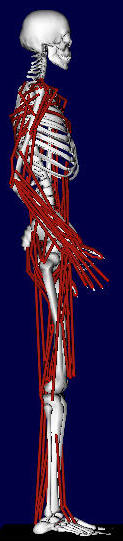Photo courtesy of OpenSim